Courses

# Applications of Gauss' Law - Electrostatics, Electromagnetic Theory, CSIR-NET Physical Sciences Physics Notes | EduRev

## Physics for IIT JAM, UGC - NET, CSIR NET

Created by: Akhilesh Thakur

## Physics : Applications of Gauss' Law - Electrostatics, Electromagnetic Theory, CSIR-NET Physical Sciences Physics Notes | EduRev

The document Applications of Gauss' Law - Electrostatics, Electromagnetic Theory, CSIR-NET Physical Sciences Physics Notes | EduRev is a part of the Physics Course Physics for IIT JAM, UGC - NET, CSIR NET.
All you need of Physics at this link: Physics

Gauss’s law is useful incalculating the electric field in problems, in which it is possible to choose a closed surface such that the electric field has a normal component which is either zero or has a single fixed (constant) value at every point on the surface. Such a closed surface, for a given charge distribution, is referred to as the ‘Gaussian Surface’ for that charge distribution. We can normally find such a Gaussian surface only for charge distributions that have a high degree of symmetry associated with them. It is only when we are able to think of a suitable Gaussian surface (for a given charge distribution) that we are able to use Gauss’s theorem for a simple calculation of electric fields.

B.1.1  Electric Field due to a point charge (Coulomb’s Law)

We have considered Coulomb’s law as fundamental equation of electrostatics and have derived Gauss’s law from it. However Coulomb’s law can also be derived from Gauss’s law. This is done by using this law to obtain the expression for the electric field due to a point charge.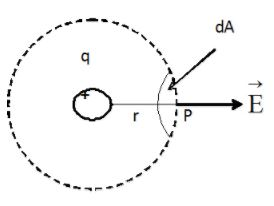Figure 3B.1 Electric field at a point on the spherical Gaussian surface surrounding a point charge.

Consider the electric field due to a single positive point charge q. By symmetry, the field is everywhere  radial and its magnitude is the same at all points, that are at the same distance r from the charge as shown in figure 3B.1. Hence, if we select, as a Gaussian surface, a spherical surface of radius r,  at all points on this surface and the field is radial. If we consider a small elementary area of this gaussian surface, the area vector is in the radial direction i.e. perpendicular to surface. Then.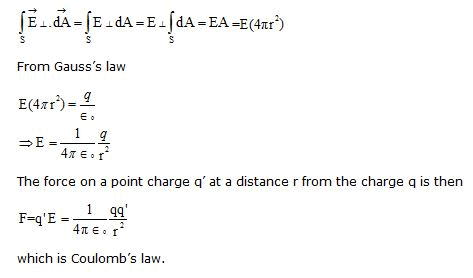Gauss’s law is a direct consequence of the inverse square nature of Coulomb’s law. The two laws can be viewed as two different ways of expressing the same basic fact-the inverse square nature of the force between two point charges. This also implies that we can think of a ‘Gauss’s law’ for any force field (like the Gravitational force) that has an ‘inverse square’ nature.

B.1.2. Electric Field due to a uniformly charged sphere

A spherically symmetric distribution of charge means the distribution of charge where the charge density ρ depends only on the distance of the point from the center and not on the direction. Let the spherically symmetric charge distribution be characterised by a charge density function, ρ(r), which varies in a certain manner, with distance, from the center of the spherical surface. Consider first the case of a charge q that is uniformly distributed over a sphere of radius R. Let us calculate the electric field strength at any point distant r from the centre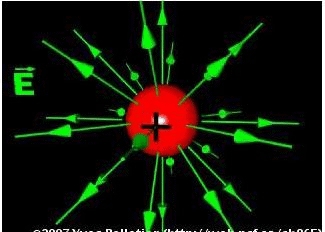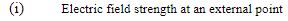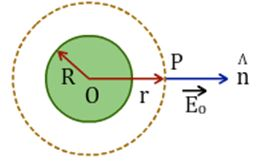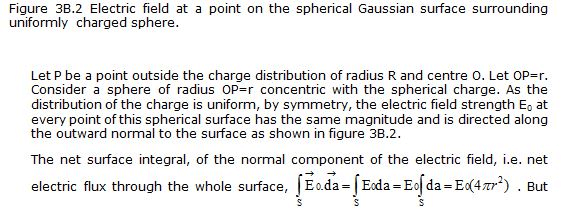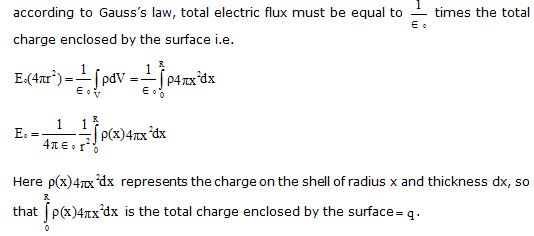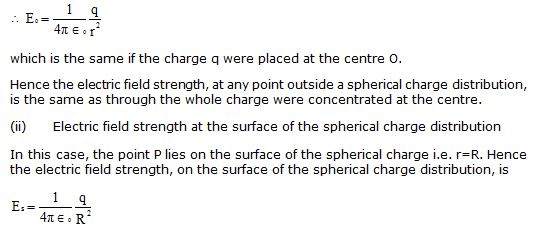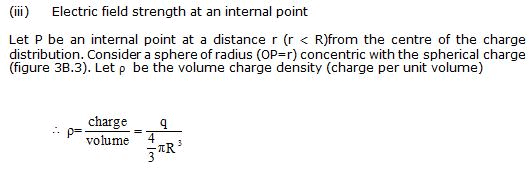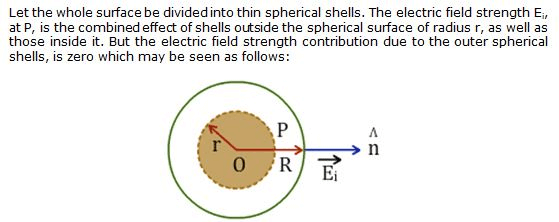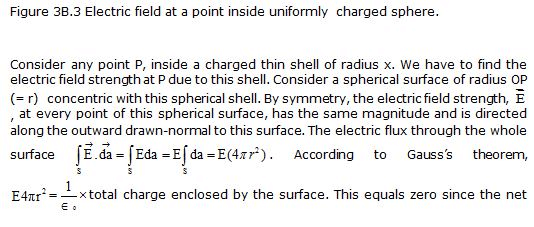charge enclosed by this internal surface is zero. This implies that E=0.

Thus electric field strength, due to a charged spherical shell, at an internal point is zero. Hence the electric field strength, Ei, at P, is due to the inner shells only, which may be found as follows:

By symmetry, the electric field strength Ei at every point of the spherical surface of radius r has the same magnitude and is directed along the outward drawn normal to the surface. The total electric flux through the whole surface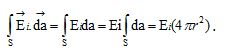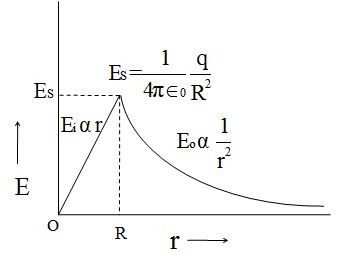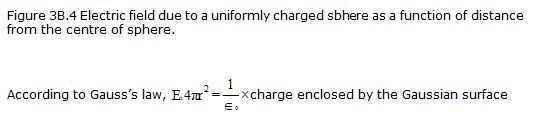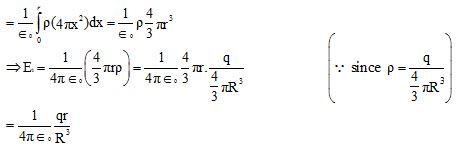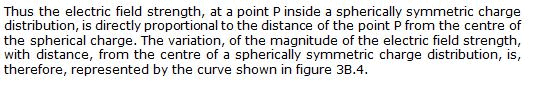159 docs

,

,

,

,

,

,

,

,

,

,

,

,

,

,

,

,

,

,

,

,

,

,

,

,

,

,

,

;